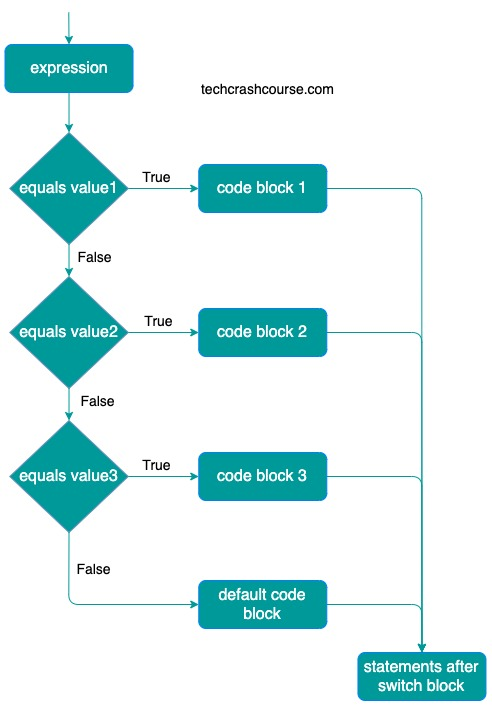# Switch Case Statement in Java

A switch statement in java allows us to selectively execute a block of statements based on the value of a variable or constant expression. In a switch case statement a variable or value of an constant expression is tested for equality against a list of possible case labels and when match is found, the block of code associated with matched case is executed.

It is similar to if..else ladder statement, but the syntax of switch statement is more readable and easy to understand.

## Syntax of Switch Statement in Java

```switch(expression) {
case value1 :
statements; // Code block 1
break;
case value2 :
statements; // Code block 2
break;
case value3 :
statements; // Code block 3
break;
default :
statement; // Default code block
}
```

First of all, expression is evaluated and it's value is compared with the values of each case.

• If the value of expression equals to value1, the code of case value1 will be executed.
• If the value of expression equals to value2, the code of case value2 will be executed.
• If the value of expression equals to value3, the code of case value3 will be executed.
• If the value of expression doesn't match wull any case values then the default code block will be executed.## Java Switch Case Statement Example Program

```public class SwitchCaseStatement {
public static void main(String[] args) {
int a = 10, b = 5;
char operator = '*';

System.out.println("a = " + a + ", b = " + b);
switch (operator) {
case '+':
System.out.println("a+b = " + (a + b));
break;
case '-':
System.out.println("a-b = " + (a - b));
break;
case '*':
System.out.println("a*b = " + (a * b));
break;
case '/':
System.out.println("a/b = " + (a / b));
break;
default:
System.out.println("Invalid Operator");the
}
}
}
```
Output
```a = 10, b = 5
a*b = 50
```

In above program, we are using switch case statement to perform arithmetic operation between variable a and b based on value of character variable operator. The value of the operator variable will be compared with each case value and the code block of the matching case will execute.

In above example, the value of operator variable is "*", which matches with the third case value and the product of a and b is printed.

### Important points about Switch Case Statement

• The expression in switch case must evaluates to char, byte, short, int or enum.

• You can use any number of case labels within a switch. Case labels must be unique. We cannot write two case blocks with same constants.

• Switch case performs only equality check of the value of expression/variable against the list of case values.

• The default code block gets executed when none of the case matches with expression. default case is optional and doesn't require a break statement.

• The break statement is optional.The break statement at the end of each case cause switch statement to exit. If break statement is not used, all statements below that case statement are also executed until it found a break statement.

• One switch statement can have maximum of one default label. Default block can be placed anywhere in switch statement.